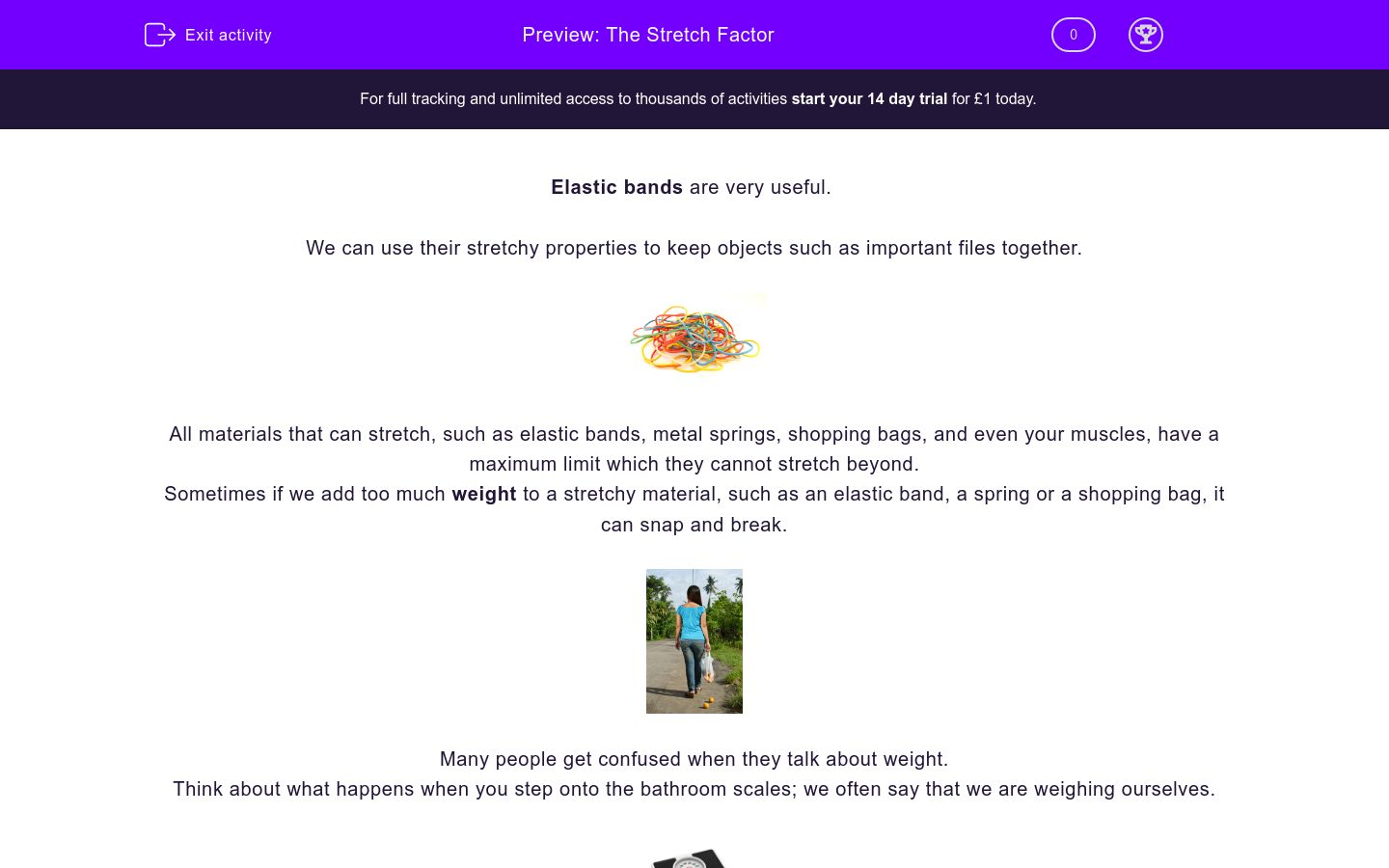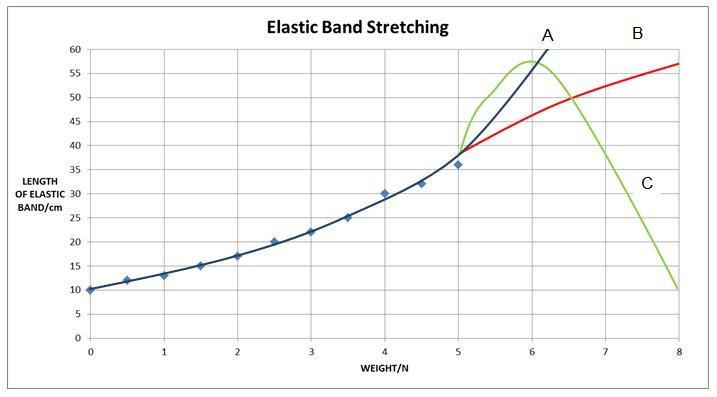# The Stretch Factor

In this worksheet, students will be challenged to use the results from an experiment to determine how an elastic material behaves and interpret the results on a graph.Key stage:  KS 3

Curriculum topic:   Working Scientifically

Curriculum subtopic:   Analysis and Evaluation

Difficulty level:### QUESTION 1 of 10

Elastic bands are very useful.

We can use their stretchy properties to keep objects such as important files together.All materials that can stretch, such as elastic bands, metal springs, shopping bags, and even your muscles, have a maximum limit which they cannot stretch beyond.

Sometimes if we add too much weight to a stretchy material, such as an elastic band, a spring or a shopping bag, it can snap and break.Many people get confused when they talk about weight.

Think about what happens when you step onto the bathroom scales; we often say that we are weighing ourselves.Unfortunately this statement is not scientifically correct. The number we have just obtained is our mass, not our weight.

The reason why is because weight is a force and should be measured in Newtons (N) not kilograms (or pounds or stones).

To convert mass (in kilograms) into Newtons you need to multiply mass by 10.

In this worksheet you get the chance to find out more about mass and weight, and test an elastic band to its limits!

Elastic bands and springs are good examples of elastic materials because when a force is applied to them and then released, what do they do?stay as they are

stretch

When we measure our mass on a set of bathroom scales we get a number in kilograms (kg).Remember, this number is our mass, not our weight.

To convert mass (in kilograms) into Newtons you need to multiply mass by 10.

This is because weight can be calculated using the special formula:

weight = mass × gravity

On Earth, gravity is given a value of 10 N.

Let's practise:

My mass is 85 kg; how much do I weigh?

Weight = 85 x 10

Weight = 850 N

​Now use the rule you have just learned to match up the correct rows in the table. Either match the mass with the correct weight or the correct answer with the correct sum.

## Column B

45 kg
34 x 10
21 N
450 N
340 N
2.1 kg
0.21 x 10
2.1 N

Some young scientists were testing an elastic band to see how it behaved when they added weights to it.

Here are their results:

Weight (N) Length of the band (cm)
0 10
0.5 12
1.0 13
1.5 15
2.0 17
2.5 20
3.0 22
3.5 25
4.0 30
4.5 32
5.0 36

Which of the following statements best describe these results?

The longer the elastic band, the more weights are added.

As more weight is added, the elastic band stretches.

As the weight increases, the length of the elastic band increases.

Here are their results again, but with an extra column added showing you how much further the band stretched each time weight was added:

 Weight (N) Length of the band (cm) Increased length / Stretch (cm) 0 10 0 0.5 12 2 1.0 13 1 1.5 15 2 2.0 17 2 2.5 20 3 3.0 22 2 3.5 25 3 4.0 30 5 4.5 32 2 5.0 36 4

When the weight increased from 3.5 N to 4.0 N the band stretched an extra 5 cm.

Between 0 N and 3 N the length of the elastic band changes from 10 cm to 22 cm.

Can you work out the average amount the band stretches each time another 0.5 N weight is added between 0 N and 3 N?

3 cm

2 cm

1 cm

The young scientists then plotted a line graph of their results:Looking at it carefully, what do you think the graph shows?

The weight increases with the length of the elastic band.

The length of the elastic band increases as the weight increases.

The length of the elastic band changes with weight.

Molly, one of the young scientists, drew a line through the points like this:In what way could you criticise how Molly has joined the points?

She ought to have drawn a straight line.

She's just joined the dots together.

Her line follows all the points - it should go through the middle of them.

Max drew his line like this:Which of the following statements best describes what Max has tried to do?

He has tried to draw a straight line.

He has drawn a 'line of best fit' through as many of the points as possible.

Max has drawn a curving line up the graph.

Look at this graph:Imagine that the young scientists carried on hanging weights on to their elastic band. Which of the three possible lines do you think is the most likely?

C

B

A

If they took all of the 8 N off the elastic band, what do you predict would have happened to its length?

It would be longer than when it started.

It would go to 0 cm.

It would go back to its original length.

If the young scientists had kept adding weights to the elastic band, what do you predict would happen to it?

It would snap.

It would keep stretching until it reached its limit and then stop.

It would get thinner but it would not break.

• Question 1

Elastic bands and springs are good examples of elastic materials because when a force is applied to them and then released, what do they do?EDDIE SAYS
If you apply a force to an elastic material it will either squash or stretch. Elastic materials can change shape and then return to their original shape when you release them; try it with a bouncy ball.
• Question 2

When we measure our mass on a set of bathroom scales we get a number in kilograms (kg).Remember, this number is our mass, not our weight.

To convert mass (in kilograms) into Newtons you need to multiply mass by 10.

This is because weight can be calculated using the special formula:

weight = mass × gravity

On Earth, gravity is given a value of 10 N.

Let's practise:

My mass is 85 kg; how much do I weigh?

Weight = 85 x 10

Weight = 850 N

​Now use the rule you have just learned to match up the correct rows in the table. Either match the mass with the correct weight or the correct answer with the correct sum.

## Column B

45 kg
450 N
21 N
2.1 kg
340 N
34 x 10
0.21 x 10
2.1 N
EDDIE SAYS
It's a really important rule, and one that you will learn a lot more about in the future.
Weight is a force, measured in Newtons: it can be calculated by multiplying the mass of an object by gravity (which on Earth has a value of 10).
• Question 3

Some young scientists were testing an elastic band to see how it behaved when they added weights to it.

Here are their results:

Weight (N) Length of the band (cm)
0 10
0.5 12
1.0 13
1.5 15
2.0 17
2.5 20
3.0 22
3.5 25
4.0 30
4.5 32
5.0 36

Which of the following statements best describe these results?

As the weight increases, the length of the elastic band increases.
EDDIE SAYS
It's true that the band stretches as weight is added, but it's a better description of the results to say that the length increases as the weight increases - we call that being 'proportional'.
• Question 4

Here are their results again, but with an extra column added showing you how much further the band stretched each time weight was added:

 Weight (N) Length of the band (cm) Increased length / Stretch (cm) 0 10 0 0.5 12 2 1.0 13 1 1.5 15 2 2.0 17 2 2.5 20 3 3.0 22 2 3.5 25 3 4.0 30 5 4.5 32 2 5.0 36 4

When the weight increased from 3.5 N to 4.0 N the band stretched an extra 5 cm.

Between 0 N and 3 N the length of the elastic band changes from 10 cm to 22 cm.

Can you work out the average amount the band stretches each time another 0.5 N weight is added between 0 N and 3 N?

2 cm
EDDIE SAYS
Add the amount the band stretches for each amount of weight added, then divide this by the number of times weight was added, which in this case was six.
In this example 2+1+2+2+3+2 = 12, 12/6 = 2
So it works out at an average of 2 cm per 0.5 N even though it's not always exactly a 2 cm stretch.
• Question 5

The young scientists then plotted a line graph of their results:Looking at it carefully, what do you think the graph shows?

The length of the elastic band increases as the weight increases.
EDDIE SAYS
You can see the line going steadily up to the right: as the weight hung on the band increases, so it pulls with a greater force, so the length of the elastic band increases.
• Question 6

Molly, one of the young scientists, drew a line through the points like this:In what way could you criticise how Molly has joined the points?

Her line follows all the points - it should go through the middle of them.
EDDIE SAYS
As you can see, the line of the points is not straight - it's a gentle curve, but Molly should draw a line that follows what is called the 'best fit' because in any experiment the results are not perfect and you are looking for the basic 'trend' of what happened.
• Question 7

Max drew his line like this:Which of the following statements best describes what Max has tried to do?

He has drawn a 'line of best fit' through as many of the points as possible.
EDDIE SAYS
In any science experiment it's hard to get perfect results - they're likely to be 'out' by a little bit at any rate (which is why experiments are repeated again and again to check on them), so Max's line shows the basic 'trend' of what happened to their elastic band when it stretched, rather than following their results exactly.
• Question 8

Look at this graph:Imagine that the young scientists carried on hanging weights on to their elastic band. Which of the three possible lines do you think is the most likely?

B
EDDIE SAYS
Although it's always hard to be sure what will happen (which it why you have to DO the experiment to check on your predictions) it's likely that the elastic band won't have snapped yet (line C) as it's only just starting to stretch more than normal. It's likely that it will begin to reach the limit of how much more it can stretch and so stretch less and less (line B). Line A would be right for a spring which stretches much more when it 'gives way'.
• Question 9

If they took all of the 8 N off the elastic band, what do you predict would have happened to its length?

It would be longer than when it started.
EDDIE SAYS
When an elastic material is 'overstretched' as in this case (but without it breaking/snapping), it ends up being longer than when you started; the particles inside the material have been pulled further away from each other and so the elastic band would end up a little longer than when the experiment began.
• Question 10

If the young scientists had kept adding weights to the elastic band, what do you predict would happen to it?

It would snap.
EDDIE SAYS
Eventually the elastic particles inside would part company under the enormous pulling force (they would not be able to pull back against the weight any more) and so it would snap.
---- OR ----